# Xbar and s (Xbar-s) Chart

## What are the Components of the Xbar-s Chart?

The Xbar chart (the upper chart in this figure) plots the average of individual values in a subgroup (i.e., the subgroup mean). The chart (the lower chart in the figure) plots the sample standard deviation of the individual values in the subgroup. This combined chart is sometimes referred to as Xbar-SD.

### Xbar-s Charts for a Single Characteristic

A traditional Xbar-s chart is commonly used to monitor processes where the sampling strategy calls for large sample sizes, typically of 10 or more.

For example, this sample chart (taken from InfinityQS® ProFicient™ software) highlights subgroup 9 of 20 subgroups. You can see that the average of the subgroup’s plot points is 34.02 (top chart) and the standard deviation is 2.755 (lower chart).

Scroll down to learn how to use this chart.## Automate and Simplify Control Chart Analysis

See how easy it is to access actionable information from your SPC control charts.

## How to Use the Xbar-s Chart

Use the Xbar-s chart chart when your sample size is 10 or more (n≥10). This scenario is most common when a lot of data is available (or necessary) and the data acquisition cost is low.

For example, you might use this chart for data taken from Programmable Logic Controllers (PLCs) or other automated data-collection devices. Injection molding, multihead fill operations, and continuous high-speed production lines on which many measurements can be gathered quickly and affordably are all good environments for this type of chart.

Each of the special use case examples described on this page presume a large sample size (i.e., 10 or more).

InfinityQS® software takes this chart technology to the next level by supporting multilevel Pareto charts—up to 10 levels deep.

• Very sensitive to small changes in the subgroup mean
• Standard deviation is usually a more accurate indicator of process variation than is the range

• Requires gathering large amounts of data to calculate control limits

## Decision Tree

Use the following decision tree to determine whether the Xbar-s chart is the best choice.
Scroll down to see special use examples.## Special Uses

Today, control charts are a key tool for quality control and figure prominently in Lean manufacturing and Six Sigma efforts.

### Target Xbar-s Chart

Target Xbar-s charts can help you identify changes in the average and standard deviation of a characteristic. You can measure the characteristic across part numbers, but each part number must form a separate subgroup because target values change with the part number. Set the target values at the desired center, typically the center two-sided specifications.
• Plot multiple parts or characteristics with similar variability on the same chart.
• Assess statistical control for the process as well as for each of its parts or characteristics.
• Detect very small process shifts.
• Directly plot data from gauges that are zeroed out on target values (no data transformation or coding necessary).### Short Run Xbar-s Chart

Short run charts are used for short production runs. The short run Xbar-s chart can help you identify changes in the averages and standard deviation of multiple characteristics, even those with different nominals, units of measure, or standard deviations.

• Summarize a great amount of data while still detecting small changes in process average.
• Detect the difference between process- and product-specific variabilities.
• Plot variations of multiple products, even those with differing standard deviations, nominals, or units of measure—all on one chart.### Group Xbar-s Chart

Group charts display several parameters, characteristics, or process streams on one chart. Group Xbar-s charts help you assess changes in averages and the standard deviation across measurement subgroups for a characteristic.

• Compare the variations of a variety of products or characteristics.
• See the difference between variations that are caused by changes in average and those caused by changes in the standard deviation.
• Clearly detect characteristics that are priorities for attention.### Group Target Xbar-s Chart

The group target Xbar-s chart provides information about changes in process averages and the standard deviation across multiple measurement subgroups of similar characteristics that have a common process. Part numbers and engineering nominal values can differ across these characteristics.

• Compare variations of multiple products or characteristics as well as similar characteristics with different averages.
• See the difference between variations that are caused by changes in average and those caused by changes in the standard deviation.### Group Short Run Xbar-s Chart

The group short run Xbar-s chart enables you to spot changes in the process average and standard deviation across multiple characteristics in a short run environment.

• Identify the difference between process- and product-specific variations.
• Compare variations of multiple products or characteristics.
• Analyze characteristics from a variety of parts, even those with different means, standard deviations, or units of measure.
• See the difference between variations that are caused by changes in average and those caused by changes in the standard deviation.## Group Short Run Xbar-s Chart Example

### Group Short Run Xbar-s Charts

Group short run Xbar-s charts enable you to spot changes in the process average and standard deviation across multiple characteristics in a limited production run. Review the following example—an excerpt from Innovative Control Charting1—to get a sense of how a group short run Xbar-chart works.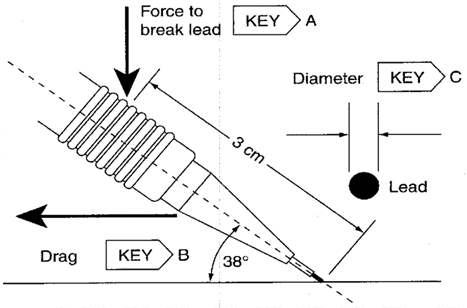Figure 1. Mechanical pencil with three key characteristics.

### Case Description

A company manufactures mechanical pencil lead. There are three key characteristics (see Table 30.5).

1. Break force—The amount of pressure it takes to break the lead (extended 1.5 mm) at a 38° angle with the force applied 3 cm from the lead rip
2. Drag—A proprietary measure of how smoothly the lead releases onto a given paper
3. Diameter—The diameter of the lead

Table 1. Upper and lower specification limits for three mechanical pencil lead key characteristics.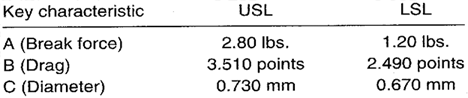The manager wishes to monitor the stability of all three key characteristics on the same chart.

### Sampling Strategy

Because production volume is very high and three different characteristics are to be monitored, a group short run Xbar-chart is selected. Ten leads are tested every 30 minutes.

### Target Values

Preliminary tests on all three key characteristics were conducted. The purpose of the tests was to establish target values for the group short run charts to be used. The target values are found in Table 2.

Table 2. Target X and target s values for the three mechanical pencil lead key characteristics.### Data Collection Sheet

Table 3. Data collection sheet for the group short run Xbar-s chart pencil lead example. MAX and MIN plot points are shown in bold.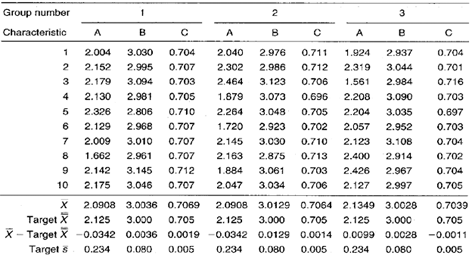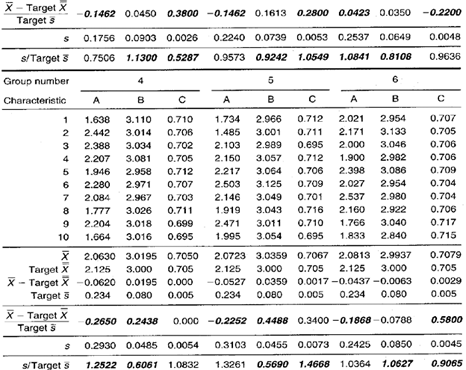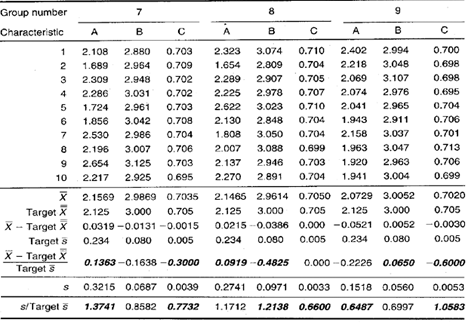### Group Short Run Xbar-s ChartFigure 2. Group short run Xbar-chart for the pencil lead example. Three key characteristics are being monitored on the same chart.

### Chart Interpretation

Group short run s chart: All three characteristics—break force (A), drag (B), and lead diameter (C)—appear to randomly fluctuate in the MAX and MIN positions. This indicates that the initial target values were good estimators for all of the characteristics.

Group short run Xbar chart: It appears that all three key characteristics are randomly fluctuating in the MAX and MIN positions. This means that the initial target values were good estimators of the actual means for each of the three characteristics.

### Recommendations

Group short run s chart: Continue using the initial target s values for all three characteristics. The charts may look good, but only the capability studies will determine if the characteristics are meeting engineering requirements.

Group short run Xbar chart: Continue using the initial target X values. No recalculation is necessary. The process averages appear stable and predictable. Continue to collect data. If the process remains stable, reduce sampling frequency.

### Estimating the Process Average

Estimates of the process average should be calculated separately for each characteristic on each part on the group short run charts. The estimate of the process average for break force can be found in Calculation 1.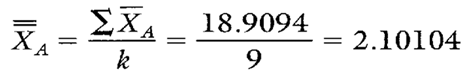Calculation 1. Estimate of the process average for characteristic A, break force.

### Estimating Sigma

Estimates of sigma are also calculated separately for each characteristic on each part on the group short run charts. Continuing with characteristic A, see Calculations 2 and 3.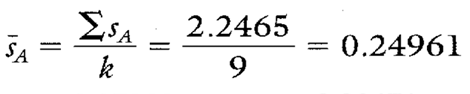Calculation 2s calculation for characteristic A, break force.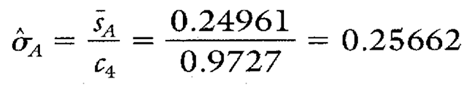Calculation 3. Estimate of the process standard deviation for characteristic A, break force.

Note: To ensure reliable estimates of both the process average and process standard deviation, k needs to be at least 20. In this example, k is only nine. Therefore, the estimates here and in Table 4 are shown only for illustration purposes.

### Calculating Process Capability and Performance Ratios

Calculations 4, 5, and 6 show the capability calculations for break force, characteristic A.Calculation 4. Cp calculation for characteristic A, break force.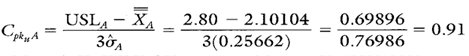Calculation 5. Cpk upper for characteristic A, break force.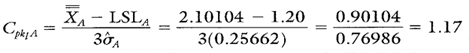Calculation 6. Cpk lower calculation for characteristic A, break force.

### Group Short Run Xbar-s Chart Advantages

• Graphically illustrates the variation of multiple product or process characteristics relative to each other.
• Characteristics from different parts with different means, different standard deviations, and different units of measure can all be analyzed on the same chart.
• Separates variation due to changes in the average from variation due to changes in the standard deviation.
• Separates variation due to the process from variation that is product specific.

### Group Short Run Xbar-s Chart Disadvantages

• No visibility of characteristics that fall between the MAX and MIN plot points
• Cannot detect certain nonrandom conditions because the group charts described here have no control limits
• Lots of calculations

Additional statistics and process capability and performance calculations for key characteristics B and C are shown in Table 4.

Table 4. Additional statistics and process capability and performance calculations for the drag and diameter key characteristics.When you use SPC software from InfinityQS, consuming the information provided by group short run Xbar-charts becomes faster and easier than ever. See how this type of analysis is surfaced in InfinityQS solutions.

FOOTNOTE:
1 Wise, Stephen A. and Douglas C. Fair. Innovative Control Charting: Practical SPC Solutions for Today’s Manufacturing Environment. Milwaukee, WI: ASQ Quality Press.

## Group Target Xbar-s Charts

Group target Xbar-s charts provide information about changes in process averages and the standard deviation across multiple measurement subgroups of similar characteristics that have a common process. Review the following example—an excerpt from Innovative Control Charting1—to get a sense of how a group target Xbar-chart works.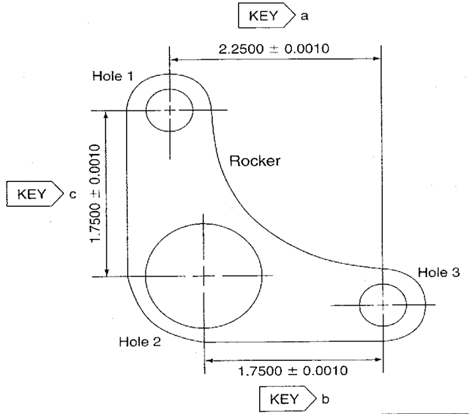Figure 1. Three hole-location measurements from a rocker.

### Case Description

The rocker shown in Figure 1 is machined from an iron casting. There have been complaints from field mechanics that the rockers are not interchangeable and that the holes do not always line up with mating parts. To monitor the uniformity of the hole locations, the operators would like to use a chart at the milling machine to track the variability of the three hole locations.

### Sampling Strategy

Because production volume is very high and all the measurements represent hole locations of different distances created on the same machine, a group target Xbar-chart is selected. Ten rockers are measured every hour.

### Data Collection Sheet

Table 1. Group target Xbar-s chart data collection sheet for three hole locations on a rocker. MAX and MIN plot points are shown in bold.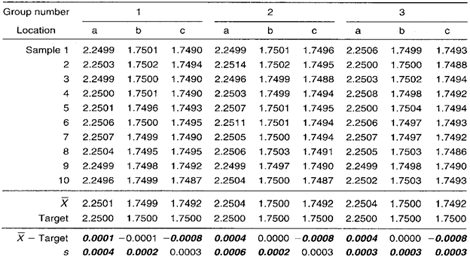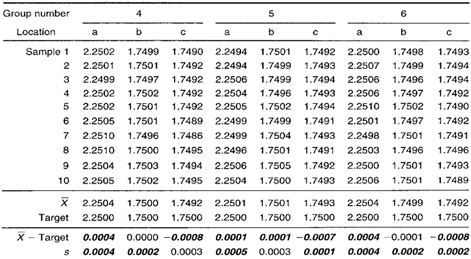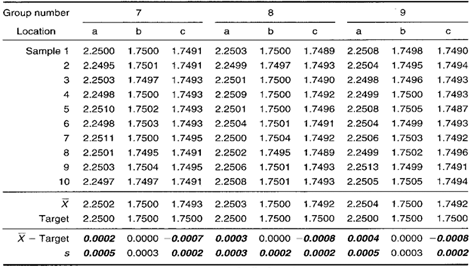### Group Target Xbar-s ChartFigure 2. Group target Xbar-chart representing three different hole locations on the same part.

### Chart Interpretation

Group s chart: Location a appears in the MAX position in every group. This indicates that location a has the largest standard deviation. Locations b and c appear randomly in the MIN position, meaning that location b and c’s standard deviation values are both similar to one another and smaller than location a’s.

Note: The centerline on the group s chart is the average of all the sample standard deviation values on the data collection sheet.

Group target Xbar chart: The coded Xbar for location a appears in the MAX. position in every group and its value is always positive. This indicates that the average hole location at location a is consistently higher than the engineering nominal (target) value.

Location appears in the MIN position in all nine groups and its value is always negative. This means that the average hole location distance at location c is consistently lower than its engineering nominal (target) value.

Note: The centerline on the group target Xbar chart is the average of all the coded Xbar plot points in the data collection sheet.

### Recommendations

• The group target Xbar chart reveals two consistent problems: Location a is always wider than target, and location c is always closer. This type of problem is fixed by changing the location of one or more holes during the job setup. The chart itself does not indicate which hole to relocate. A logical place to begin investigation is with hole 1 because its location affects both key locations a and c.
• Looking at the group s chart, the distance between holes 1 and 3 (hole location a) varies more than the other hole relationships. This also means there is excess variation in the horizontal axis. Operators should verify this assumption with process engineers and remedy the problem..

### Estimating the Process Average

If all of the locations on the group target Xbar chart were behaving randomly, a single estimate of the process average could be used to estimate the process average for all locations. However in this case, the group target Xbar chart does not exhibit random behavior.

Given nonrandom patterns on a group target Xbar chart, estimates of the process average should be calculated separately for each characteristic or location. This is illustrated in Calculation 1 using data from hole location a.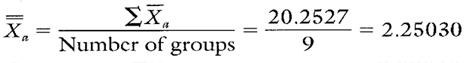Calculation 1. Estimate of the process average for hole location a.

### Estimating Sigma

Estimates of sigma are also calculated separately for each characteristic or location on the group target chart. Continuing with hole location a, see Calculations 2 and 3.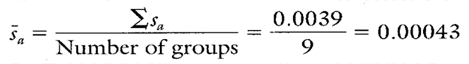Calculation 2. Calculation of for use in estimating the process standard deviation for hole location a.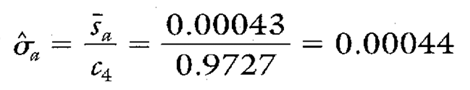Calculation 3. Estimate of the process standard deviation for hole location a.

Note: To ensure reliable estimates, the number of groups should be at least 20. In this example, the number of groups is only nine. Therefore, the estimates here and in Table 2 are for illustration purposes only.

### Calculating Process Capability and Performance Ratios

The Cp and Cpk calculations for hole location a are shown in Calculations 4, 5, and 6.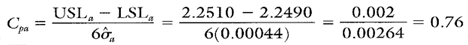Calculation 4. Cp calculation for hole location a.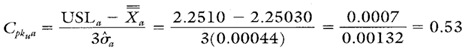Calculation 5. Cpk upper calculation for hole location a.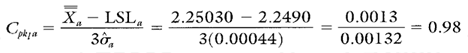Calculation 6. Cpk lower calculation for hole location a.

### Group Target Xbar-s Chart Advantages

• Simultaneously illustrates the variation of multiple product or process characteristics.
• Similar characteristics with different averages can be analyzed on the same chart.
• Separates variation due to changes in the average from variation due to changes in the standard deviation.
• Multiple characteristics can be tracked on one chart.

### Group Target Xbar-s Chart Disadvantages

• No visibility of the characteristics that fall between the MAX and MIN plot points.
• The use of negative numbers can be confusing.
• Cannot detect certain nonrandom conditions because the group target charts described here have no control limits.

The process capability and performance values for hole locations b and c are shown in Table 2.

Table 2. Summary statistics and process capability and performance ratios for hole locations and c.When you use SPC software from InfinityQS, consuming the information provided by group target Xbar-charts becomes faster and easier than ever. See how this type of analysis is surfaced in InfinityQS solutions.

FOOTNOTE:
1 Wise, Stephen A. and Douglas C. Fair. Innovative Control Charting: Practical SPC Solutions for Today’s Manufacturing Environment. Milwaukee, WI: ASQ Quality Press.

## Group Xbar-s Chart Example

### Group Xbar-s Charts

Group Xbar-s charts help you assess changes in averages and the standard deviation across measurement subgroups for a characteristic. Review the following example—an excerpt from Innovative Control Charting1—to get a sense of how a group Xbar-chart works.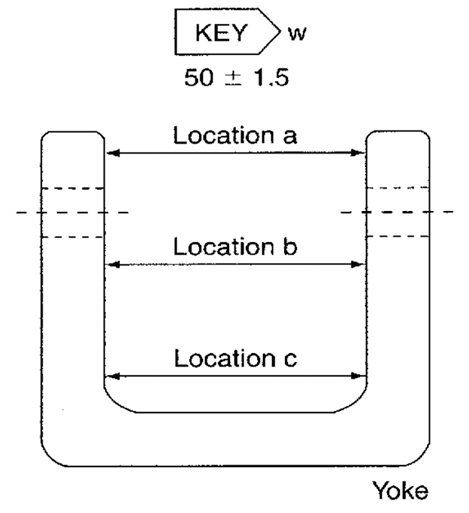Figure 1. Three width measurements from a yoke.

### Case Description

This yoke is machined from an aluminum casting. There have been complaints from the assembly department that some of the yokes have a taper on the inside width. To monitor the uniformity of the inside widths, a group chart is set up at the milling machine to track the width at locations a, b, and c.

### Sampling Strategy

Because the production volume is very high, and the same characteristic is being measured at three different locations on the part, a group Xbar-s chart is selected. Ten yokes are measured every hour.

### Data Collection Sheet

Table 1. Data collection sheet for the group Xbar-s chart. MAX and MIN plot points are shown in bold.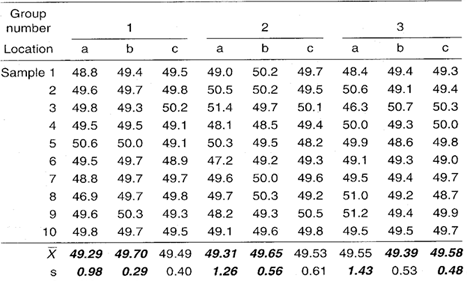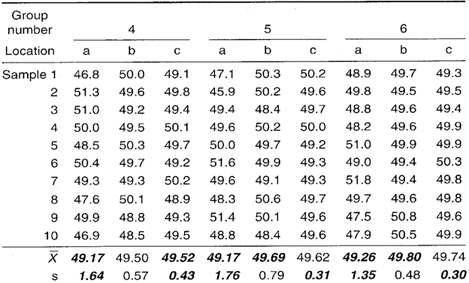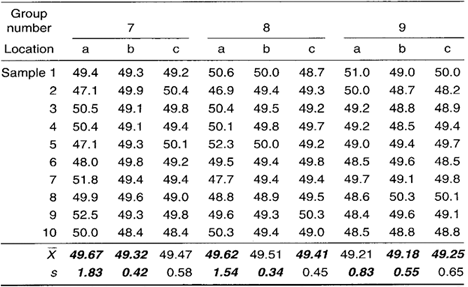### Group Xbar-s Chart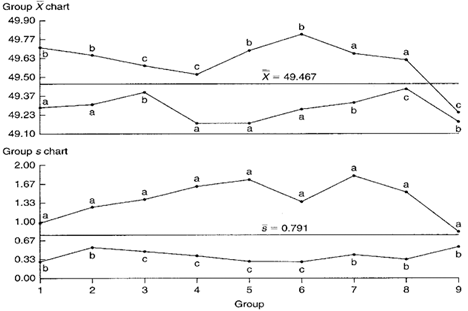Figure 2. Group Xbar-s chart representing three different yoke width locations.

### Chart Interpretation

Group s chart: Location a appears in the MAX position for all groups. This suggests that location a has the largest standard deviation. Locations b and c appear randomly in the MIN position. This indicates that locations b and c have similar standard deviations and they are less than location a’s.

Note: The centerline on the group s chart is the average of all the 5 values on the data collection sheet.

Group Xbar chart: The difference between the MAX and MIN for each group represents taper within the yokes. Locations a, b, and c appear randomly in the MAX position. However, location a appears five out of nine times in the MIN position. This might indicate that location a has a smaller diameter than either of the two other locations. However, this supposition is not as strong as it would be if location a represented the MIN position for all groups.

Note: The centerline on the group Xbar chart is the average of all the Xbar plot points found on the data collection sheet.

### Recommendation

The repeated presence of location a in the MAX position in the group s chart may be the result of the inability of tooling to hold the work piece consistently during the manufacturing of the yokes. Notice that location a is found at the end of the yoke. This may signify the need for tooling changes that will hold the outer ends more rigidly during manufacturing.

### Estimating the Process Average

Process average estimates should be performed separately for each characteristic or location on the group chart (see Calculation 1).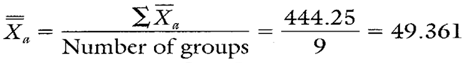Calculation 1. Estimate of the process average for yoke width at location a.

### Estimating Sigma

Estimates of sigma are also calculated separately for each characteristic or location on the group chart. Continuing with yoke width location a, see Calculations 2 and 3.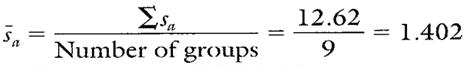Calculation 2. Calculation of the average sample standard deviation for yoke width location a.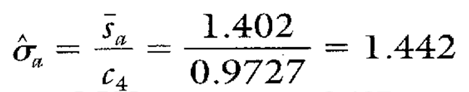Calculation 3. Estimated standard deviation for yoke width location a.

Note: To ensure reliable estimates, the number of groups should be at least 20. In this example, the number of groups is only nine. Therefore, these estimates and those found in Table 2 are only for illustration purposes.

### Calculating Process Capability and Performance Ratios

Calculations 4, 5, and 6 show the process capability and performance calculations for yoke width location a.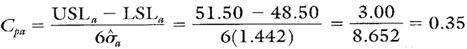Calculation 4. Cp calculation for width location a.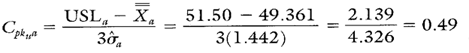Calculation 5. Cpk upper calculation for width location a.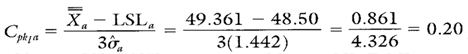Calculation 6. Cpk lower calculation for width location a.

• Graphically illustrates the variation of multiple product or process characteristics simultaneously and relative to each other.
• Pinpoints the characteristics that are in need of the most attention.
• Separates variation due to changes in the average from variation due to changes in the standard deviation.
• Multiple measurement locations can be tracked on one chart.

• No visibility of the characteristics that fall between the MAX and MIN plot points.
• Cannot detect certain out-of-control conditions because the group charts described here have no control limits.
• Given the large amounts of data used in charts, efficient analysis typically requires software.

The process capability and performance ratio calculations for yoke widths at locations b and are shown in Table 2.

Table 2. Summary statistics and process capability and performance ratios for yoke widths at locations b and c.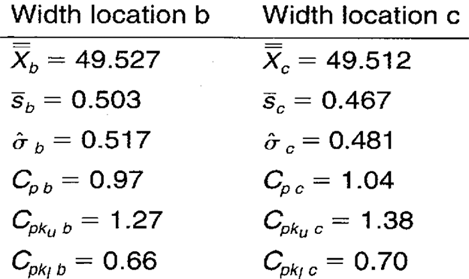When you use SPC software from InfinityQS, consuming the information provided by group Xbar-charts becomes faster and easier than ever. See how this type of analysis is surfaced in InfinityQS solutions.

FOOTNOTE:
1 Wise, Stephen A. and Douglas C. Fair. Innovative Control Charting: Practical SPC Solutions for Today’s Manufacturing Environment. Milwaukee, WI: ASQ Quality Press.

## Short Run Xbar-s Charts

Short run Xbar and s (Xbar-s) charts can help you identify changes in the averages and standard deviation of multiple characteristics in a limited production run. Review the following example—an excerpt from Innovative Control Charting1—to get a sense of how a short run Xbar-chart works.Figure 1. Delta torque is a performance key characteristic on self-locking fastener systems.

### Case Description

Torque is tested on self-locking nuts using precision stud standards and production nuts. During production, the nuts are slightly deformed so that the threads create an interference or locking fit with the stud. The run-on torque is the average prevailing torque while turning the nut on the stud seven clockwise revolutions. The runoff torque is the maximum force it takes to turn the nut back off the stud one counterclockwise revolution. The delta torque is the run-on torque minus the run-off torque. Each fastening system has its own minimum delta torque requirements and the standard deviations are expected to vary from system to system.

### Sampling Strategy

Torque tests are performed for each batch of locking nuts. Ten samples are tested from each batch. To monitor the delta torque consistency, regardless of the nut/bolt locking system, a short run Xbar-chart is selected. This is the appropriate chart because the subgroup sizes are large and the standard deviations are different from system to system.

### Target Values

Before a short run chart can be used, target values must first be defined.

### Locking System A

System A has previously been maintained using traditional Xbar-charts. On the most recent set of in-control charts, the centerline on the Xbar chart was 2.920. The centerline on the chart was 0.089. Therefore, these centerlines are used as target values for system A.Figure 2. Target values for locking system A.

### Locking System B

The consistency of locking system B has never been evaluated with a control chart. However, quality assurance personnel have taken 28 delta torque measurements at some time in the past. Equation 15.14 was used to convert the sample standard deviation from those 28 measurements into the targets found in Figure 3.Figure 3. Target values for locking system B.

### Locking System C

Like system A, Rocking system C has previously been evaluated using traditional Xbar-charts. On the most recent set of in-control charts, the centerline on the Xbar chart was 5.125. The centerline on the s chart was 0.337. Therefore, these centerlines are used as target values for system C (see Figure 4).Figure 4. Target values for locking system C.

### Data Collection Sheet

Table 1. Delta torque data sheet and plot point calculations.### Short Run Xbar-s ChartFigure 5. Delta torque short run Xbar and s control charts for locking systems A, B, and C.

### Chart Interpretation

Short run chart: If evaluating product-specific variation, locking system A’s delta torque seems to be behaving randomly. All eight of system B’s plot points fall above the centerline with one of them falling above the UCL. System C’s delta torque favors the high side with one plot point beyond the UCL. Overall, the process reveals a run of 9 plot points above the centerline that occur across three product lines (subgroups 13 through 20).

Short run Xbar chart: All seven of system A’s plot points fall below the centerline with three of them falling below the LCL. Seven of system B’s eight plot points are situated above the centerline with three above the UCL. System C appears to be behaving randomly. Looking at patterns across locking systems, there is a gradual decrease in the average from plot point 6 through 12. Also, it looks as though the average has shifted higher between plot points 13 and 20.

### Recommendations

Note: Plot point patterns above and below the centerlines and beyond the control limits are present, but the action to take depends entirely on how the target values were estimated.

### Locking System A

Short run s chart: The target came from past control charts, therefore, the fact that the plot points are behaving randomly indicates that the standard deviation has not changed since data were last recorded.

Short run Xbar chart: The target X came from past charts, therefore, the run below the centerline indicates the delta torque has decreased since data were last recorded. This is an assignable cause and should be investigated. If the shift is found to be desirable, deliberate, and permanent, the target X should be recalculated based on system A’s current overall average. If the shift is found to be an unwanted condition, do not recalculate target X. Instead, eliminate the cause of the downward shift.

### Locking System B

Short run chart: The target s came from past quality assurance records. The run above the centerline, therefore, indicates that the standard deviation has significantly increased since data were last recorded. This may be an assignable cause and should be investigated. If the shift is found to be an unwanted condition, do not recalculate target s. Instead, eliminate the cause of the increased variability.

Short run Xbar chart: The target came from quality assurance records, therefore, the run above the centerline indicates the delta torque has increased since data were last recorded. This may be an assignable cause and should be investigated. If this significant increase in delta torque is desirable, then the target X should be recalculated based on system B’s current overall average. If the shift is unwanted, do not recalculate target X. Instead, eliminate the assignable cause for the increase in the delta torque average.

### Locking System C

Short run s chart: Because the target s was based on the centerline from an older, in-control s chart, the run above the centerline indicates that the process standard deviation has increased significantly since the last time the system C product was manufactured. This should be treated as an assignable cause because the target is based upon actual data. If the increase in standard deviation for system C is expected to be a permanent change, then the target should be recalculated based on the current overall average standard deviation (see Calculation 1). Otherwise, if the assignable cause is to be removed to reduce the current amount of variation, the old target should be saved to represent the current expected level of variability.Calculation 1. Recalculating locking system C’s target s based on current data from control chart. This is done only if the change in variability is expected to be a permanent one.

Short run Xbar chart: The target has been obtained from a recent in-control chart, and the plot points are behaving randomly. This indicates that the initial target X was a good estimator of the actual delta torque. There is no need to recalculate system C’s target X.

### Estimating the Process Average

Estimates of the process average should be calculated separately for each characteristic or part on short run Xbar-s charts. In this case, estimates of the process average should be calculated separately for each different locking system. Calculation 2 shows the calculation for the estimate of the overall average of locking system B.Calculation 2. Estimate of the process average for locking system B.

### Estimating Sigma

Estimates of sigma are also calculated separately for each characteristic or location represented on short run Xbar-s charts. In this case, estimates of the process standard deviation should be calculated for each different locking system. Estimates of the process standard deviation for locking system B are found in Calculation 3.Calculation 3. Calculation of for locking system B based on current data from the short run s control chart.Calculation 4. Calculation of the estimate of the process standard deviation for locking system B.

Note: To ensure reliable estimates, k needs to be at least 20. In this example, k is only 8. Therefore, the estimates here and in Table 2 are used for illustration purposes only.

### Calculating Process Capability and Performance Ratios

The Cpk lower calculation for locking system B is shown in Calculation 5. Because there is only a minimum specification, no Cp or Cpk upper value is calculated for locking system B.Calculation 5. Cpk lower calculation for fastener system B delta torque.

### Short Run Xbar-s Chart Advantages

• Graphically illustrates the variation of multiple products with different nominals, different standard deviations, and even different units of measure all on the same chart.
• Separates sources of process variability from sources of product variability.
• Due to the large sample sizes, the short run Xbar chart is sensitive to small changes in the process average.
• Summaries large amounts of data.

### Short Run Xbar-s Chart Disadvantages

• Requires software to effectively handle large amounts of data.
• The use of negative numbers and unitless ratios may be confusing at first.
• X, s, and process standard deviation estimates must be calculated separately for each characteristic represented on the chart.

• The process capability and performance ratio calculations for locking systems A and C are found in Table 2.
• Summary statistics and Cpk lower values for systems A and C are based on the actual data from the data collection sheet (Table 1). In addition, no Cp or Cpk upper values are found in Table 2 because the locking systems all have one-sided specifications.

Table 2. Additional summary statistics and process performance ratios for locking systems A and C.When you use SPC software from InfinityQS, consuming the information provided by short run Xbar-charts becomes faster and easier than ever. See how this type of analysis is surfaced in InfinityQS solutions.

FOOTNOTE:
1 Wise, Stephen A. and Douglas C. Fair. Innovative Control Charting: Practical SPC Solutions for Today’s Manufacturing Environment. Milwaukee, WI: ASQ Quality Press.

## Using the Target Xbar-s Chart: Example

See how the target Xbar-chart enables plant-floor personnel to maintain tight tolerances on high-volume production lines.

## How Do You Use Target Xbar-s Charts?

Target Xbar and s (Xbar-s) charts can help you identify changes in the average and standard deviation of a characteristic. Review the following example—an excerpt from Innovative Control Charting1—to get a sense of how a target Xbar-chart works.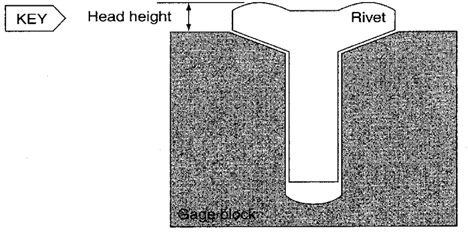Figure 1. Rivet head height is a key characteristic. The measurement is taken with the aid of a gauge block.

### Case Description

Rivet head height is a key characteristic. The height is measured off a gauge block. If the height is too low, the installed rivet will recede below the surface. If it is too high, it will protrude. Either case requires rework and is unacceptable. Three different types of rivets are manufactured, each with different target head heights and tolerances. In this example, the target Xbar-s chart allows operators to maintain extremely tight tolerances for a high-volume, high-speed production process.

### Bring SPC Charts Up to Speed

This example provides a deep dive into the manual calculations behind the target Xbar-s chart. InfinityQS® solutions—ProFicient™ and Enact®—automate chart creation and help you optimize processes faster.

Table 1. Target head heights and specifications.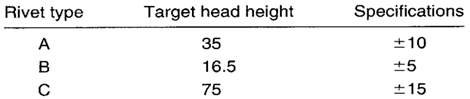### Sampling Strategy

Several rivet types are to be plotted on the same chart, but because only one characteristic, head height, is to be controlled, use of a target chart would be appropriate. The production volume is extremely high (thousands per hour), the data collection is quick, and the analysis is being done with the assistance of computer software. For all these reasons, a target Xbar-s chart is selected.

To determine how often measurements should be taken, a header mechanic is surveyed. It is revealed that adjustments to the equipment affecting head height are made about every hour. To capture the effects of these adjustments, samples of 10 are taken every 10 minutes.

### Data Collection Sheet

Table 2. Data collection sheet for three different rivet head heights.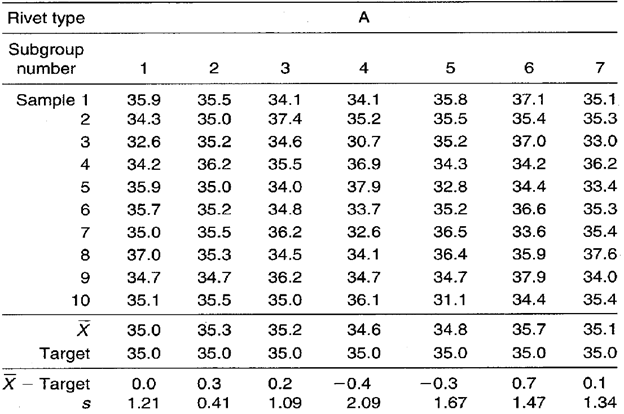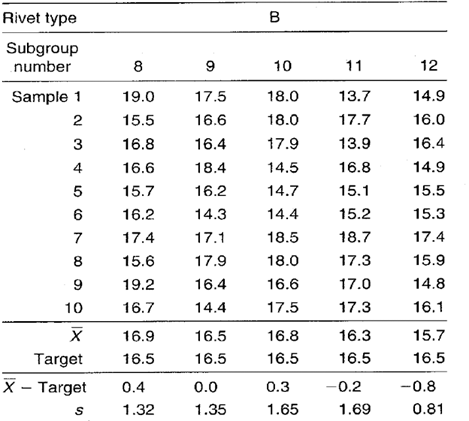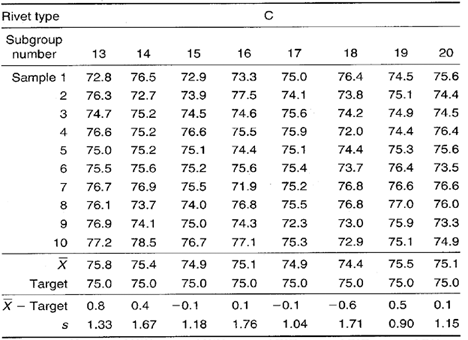### Target Xbar-s Chart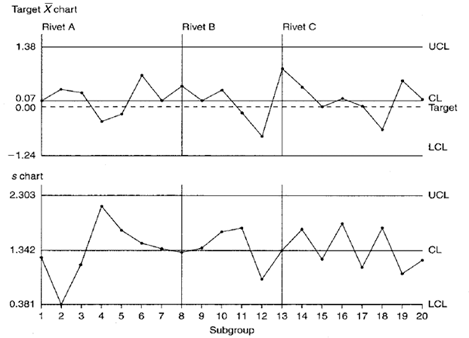Figure 2. Head height target Xbar-s control chart.

### Control Limit Calculations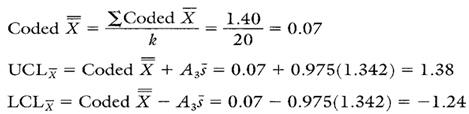Calculation 1. Calculations for target Xbar chart.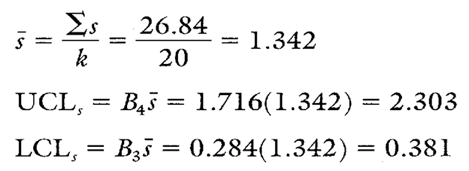Calculation 2. Calculations for s chart.

### Chart Interpretation

s chart: The chart is in control. This shows that the sample standard deviations of head heights for all three rivet types are similar.

Target Xbar chart: This chart is also in control. There are no indications of assignable causes. This means that the difference between the average head heights of all three rivet types and their respective targets is about the same.

### Recommendations

• Based on the target Xbar chart, the process is running very close to target regardless of rivet type. This is a situation where the process should not be adjusted.
• Even though the standard deviations are similar for all three rivet types, one will still need to calculate separate Cp and Cpk ratios. This is necessary because the engineering tolerances are different for each rivet type.

### Estimating the Process Average

Because the target Xbar chart is in control, the process average for all rivet types can be estimated using the coded X.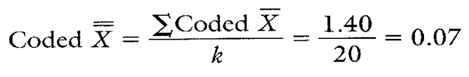Calculation 3. Estimate for the coded overall process average rivet head height (to be used in Cpk calculations for all three rivet types).

### Estimating Sigma

Because the s chart is in control, the process standard deviation can be estimated for all three rivet types using the formula found in Calculation 4.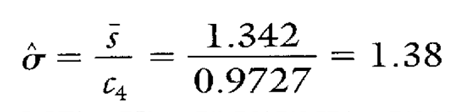Calculation 4. Estimating sigma using s.

### Calculating Process Capability and Performance Ratios

These ratios are calculated using coded data. The coded nominal for the head height characteristic is zero. Therefore, for rivet A, the coded USL is +10 and the coded LSL is –10. Following are calculations for the rivet A head height.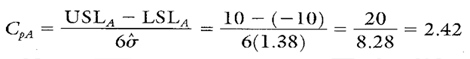Calculation 5. Cp calculation for rivet A head height.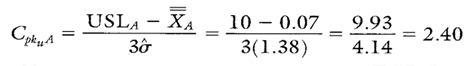Calculation 6. Cpk upper calculation for rivet A head height.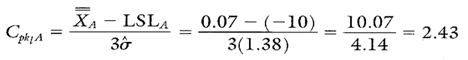Calculation 7. Cpk lower calculation for rivet A head height.

• Multiple parts or characteristics can be plotted on the same chart (provided they all exhibit similar variability).
• Data from gauges that are zeroed out on their target values can be plotted directly on the target Xbar without data coding or data transformation.
• Statistical control can be assessed for both the process and each unique part and/or characteristic being made in the process.
• Due to the large subgroup size, the Xbar chart is very sensitive to small process shifts.

• Requires software to efficiently handle the large amounts of data.
• The use of coded negative numbers can sometimes be confusing.
• When interpreting the target Xbar chart, both the zero line and the coded X must be taken into account. This accounts for some added complexity when interpreting the chart.

• Process capability and performance calculations for the B and C rivets are shown in Table 3.
• Because the target Xbar-s chart proved to be in control, the only values that change when calculating the capability ratios are the specification limits. The coded X and sigma values used to calculate Cp and Cpk ratios are the same for all three rivet types.

Table 3. Cp and Cpk calculations for B and C rivets.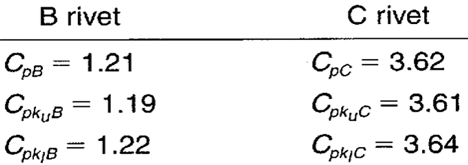FOOTNOTE:
1 Wise, Stephen A. and Douglas C. Fair. Innovative Control Charting: Practical SPC Solutions for Today’s Manufacturing Environment. Milwaukee, WI: ASQ Quality Press.

## Speak to a Manufacturing Industry Expert

What to Expect

• Free 20-minute call with a product expert
• Explore which solutions best suit your needs
• No-pressure conversation
• Get a live, personalized demo
This field is for validation purposes and should be left unchanged.